#### Casual GAMES• Casual
• Puzzle
• Strategy
• Anime
• Mature
• Racing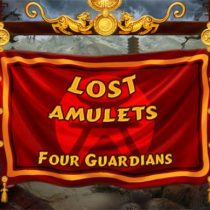• Casual
• Point & Click
• Puzzle
• Hidden Object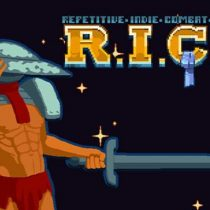• Casual
• Action• Open World
• Casual
• Hidden Object
• Building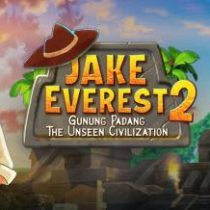• Puzzle
• Casual
• Point & Click
• Hidden Object• Simulation
• Casual
• Strategy
• RPG
• Anime
• Building• Nudity
• Casual
• Strategy
• Mature
• Puzzle
• Anime• Puzzle
• Casual
• Strategy
• Turn-Based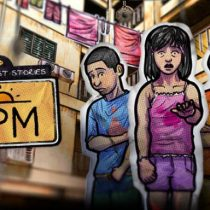• Horror
• Visual Novel
• Casual• Building
• Simulation
• Casual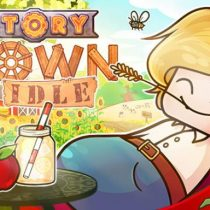• Casual
• Management
• Point & Click
• Simulation• Casual
• Puzzle
• Nudity
• Anime
• Mature• Nudity
• Casual
• Strategy
• Puzzle
• Anime• Nudity
• Anime
• Puzzle
• Casual
• Action
• Visual Novel
• Mature• Hidden Object
• Point & Click
• Casual• Casual
• Hidden Object• Nudity
• Casual
• Simulation
• Strategy
• Puzzle
• Anime